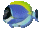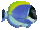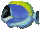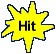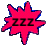Equation Word Problem Applications       Math Fun Game Tips:

- This game provides practice in forming equations for word problem applications.
1) Decide what type of number the word problem requires. Does it ask you for
the number added, the number removed, the original number, or the number remaining?
2) Use a letter variable to represent the number you are asked to find.

- In this game, you choose the correct equation to answer each word problem.
See More SliderMath (below) for games providing practice steps in solving equations.

- The word problems used in this game involve finding the ' number of ' things such as:
photos, videos, e-mails, text messages, games, songs, teams, apples, candies,...

- Your Game Score is reduced by the number of fish hits.

- To slow the game speed repeat tap/click on the word Slider.
- To increase the game speed repeat tap/click on the word Math.
- Speed can also be adjusted with a keyboard's - and + keys.

- Refresh/Reload the web page to restart the game.